## 1.1 介绍

YOLOv5 是什么？

YOLOv5 是一种流行的实时目标检测器。它是 YOLO（You Only Look Once）单次检测器的 PyTorch 实现，它以其极快的速度和合理的准确性而闻名。

YOLOv5 中可用的模型总共包含5个模型。从 YOLOv5 nano（最小最快）到 YOLOv5 extra-large（最大型号）

YOLOv5n：它是新推出的 nano 模型，是家族中最小的模型，适用于边缘、物联网设备，并且还支持 OpenCV DNN。INT8 格式小于 2.5 MB，FP32 格式约为 4 MB。它是移动解决方案的理想选择。

YOLOv5s：它是家族中的小型模型，具有大约 720 万个参数，非常适合在 CPU 上运行推理。

YOLOv5m：这是具有 2120 万个参数的中型模型。它可能是最适合大量数据集和训练的模型，因为它在速度和准确性之间提供了良好的平衡。

YOLOv5l :是 YOLOv5 家族的大型模型，有 4650 万个参数。它非常适合我们需要检测较小对象的数据集。

YOLOv5x：它是五个模型中最大的，并且在五个模型中具有最高的mAP。虽然它比其他的慢并且有 8670 万个参数。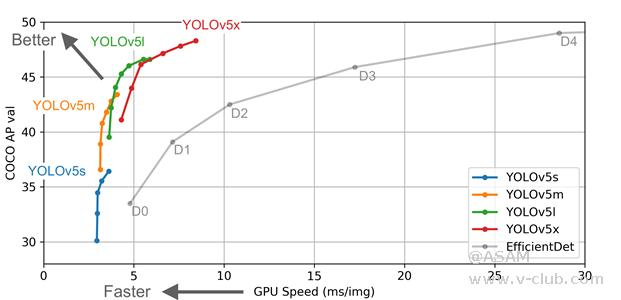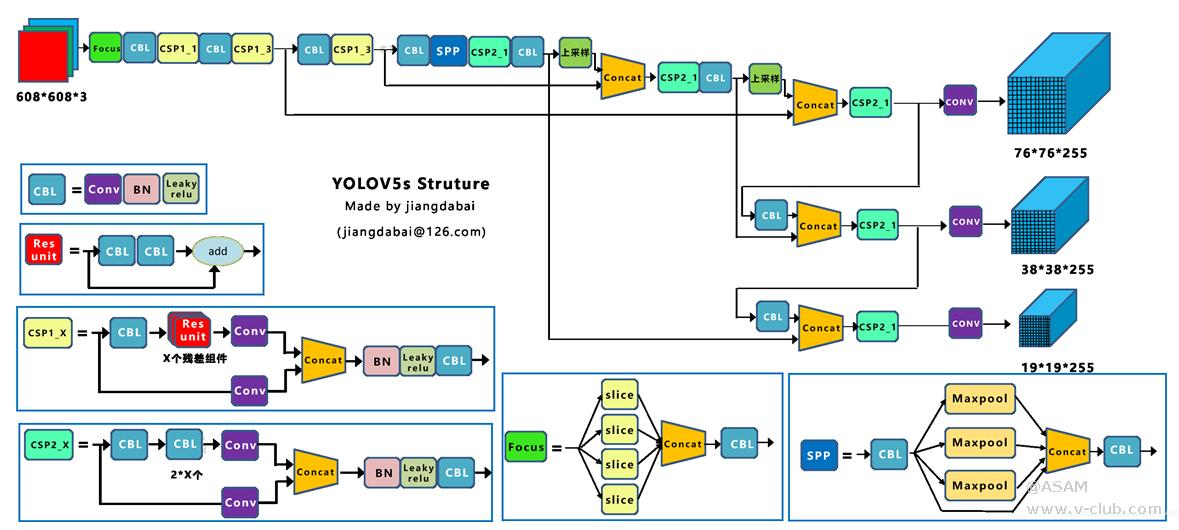但训练和推理并不是全部。它包含许多其他功能，使其非常特别。让我们回顾一下

可以将原生PyTorch (.pt)模型转换为如下格式：

TorchScript，ONNX，OpenVINO，TensorRT，CoreML，TensorFlow SavedModel, GraphDef, Lite, Edge TPU, and TensorFlow.

而我在OpenCV DNN使用的是ONNX格式。

YOLOv5的信息来自 GitHub 自述文件、问题、发布说明和 .yaml 配置文件。但是，它处于非常活跃的开发状态，我们可以期待随着时间的推移进一步改进。下表总结了 v3、v4 和 v5 的架构。

 Layers YOLOv3 YOLOv4 YOLOv5 Neural Network Type FCNN FCNN FCNN Backbone Darknet53 CSPDarknet53 (CSPNet in Darknet) CSPDarkent53 Focus structure Neck FPN (Feature Pyramid Network) SPP (Spatial Pyramid Pooling) and PANet (Path Aggregation Network) PANet Head B*(5+C) output layer B: No. of bounding boxes C: Class score 、 Same as Yolo v3 Same as Yolo v3 Loss Function Binary Cross Entropy Binary Cross Entropy Binary Cross Entropy and Logit Loss Function

Backbone：  模型主干主要提取图像的基本特征。

## 1.2使用 YOLOv5 进行目标检测训练

a.       从GitHub下载YOLOv5的源码和预训练模型（YOLOv5n, YOLOv5s, YOLOv5m, YOLOv5l, YOLOv5x）

b.      安装labelimg对照片进行标注，记得更改为yolo v5的格式。最后生成的是.xml格式记录照片标注的文件。将生成的可训练的数据集复制到和 YOLOv5 工程同一根目录下其中，YOLOv5 文件夹是工程代码，datasets 文件夹是数据集。数据集的文件目录是：其中，images 文件夹中存储的图片，labels 文件夹中存储的标签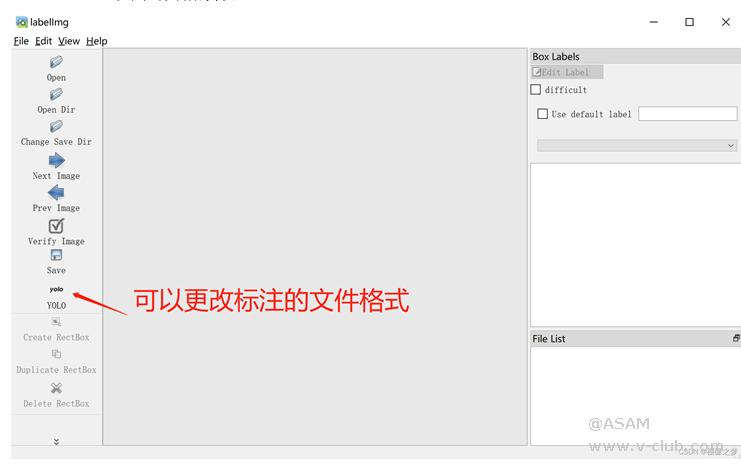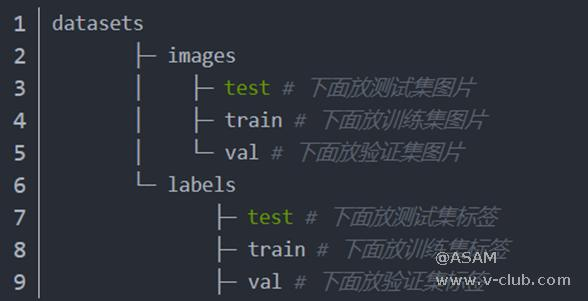c.       配置数据集文件。在 YOLOv5/data文件夹修改配置数据集文件data.yaml

# train and val data as 1) directory: path/images/, 2) file: path/images.txt, or 3) list: [path1/images/, path2/images/]

train: ../ datasets /images/

val: ../ datasets /images/

# number of classes

nc: 1

# class names

names: [thing']

d.      配置模型文件。在 YOLOv5/models 文件夹中添加一个 train.yaml

e.       运行 train.py 文件生成.pt文件，再运行export.py文件将.pt文件转换成.onnx文件。

train.py 文件和export.py文件在YOLOv5的源码中有。

## 2.1MV-EB435i立体相机介绍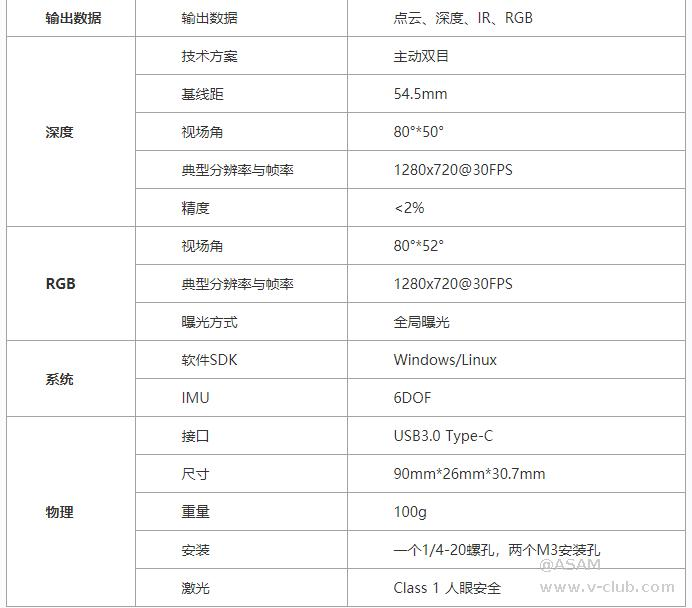## 3.1导入库与定义全局参数

``````// 常数
const float INPUT_WIDTH = 640.0f;
const float INPUT_HEIGHT = 640.0f;
const float SCORE_THRESHOLD = 0.5f;
const float NMS_THRESHOLD = 0.45f;
const float CONFIDENCE_THRESHOLD = 0.45f;

// 文本参数

cv::Mat depth_all;
const float FONT_SCALE = 0.7f;
const int FONT_FACE = FONT_HERSHEY_SIMPLEX;
const int THICKNESS = 1;
int X;
int Y;``````

SCORE_THRESHOLD：过滤低概率类分数。

NMS_THRESHOLD：删除重叠的边界框。

CONFIDENCE_THRESHOLD：过滤低概率检测。

## 3.2绘制标签

``````oid draw_label(Mat& input_image, string label, int left, int top)    //绘制标签
{
// Display the label at the top of the bounding box.
int baseLine;
Size label_size = getTextSize(label, FONT_FACE, FONT_SCALE, THICKNESS, &baseLine);
top = max(top, label_size.height);
// 左上角
Point tlc = Point(left, top);
// 右下角。
Point brc = Point(left + label_size.width, top + label_size.height + baseLine);
// 绘制黑色矩形
rectangle(input_image, tlc, brc, BLACK, FILLED);
// 将标签放在黑色矩形上。
putText(input_image, label, Point(left, top + label_size.height), FONT_FACE, FONT_SCALE, YELLOW, THICKNESS);
}``````

## 3.3预处理

``````vector<Mat> pre_process(Mat& input_image, Net& net)           //预处理
{
// 转换为 blob.
Mat blob;
blobFromImage(input_image, blob, 1. / 255., Size(INPUT_WIDTH, INPUT_HEIGHT), Scalar(), true, false);

net.setInput(blob);

// 前向传播
vector<Mat> outputs;
net.forward(outputs, net.getUnconnectedOutLayersNames());

return outputs;
}``````

## 3.4后处理

a.循环检测。

b.过滤掉好的检测。

c.获取最佳组分数的索引。

d.丢弃类别分数低于阈值的检测。

``````Mat post_process(Mat& input_image, vector<Mat>& outputs, const vector<string>& class_name)
{
// 初始化向量以在展开检测时保存各自的输出。
vector<int> class_ids;
vector<float> confidences;
vector<Rect> boxes;

// 调整大小的因素
float x_factor = input_image.cols / INPUT_WIDTH;
float y_factor = input_image.rows / INPUT_HEIGHT;

float* data = (float*)outputs.data;

const int dimensions = 85;
const int rows = 25200;
// 迭代 25200 次检测。
for (int i = 0; i < rows; ++i)
{
float confidence = data;
// 丢弃错误检测并继续。
if (confidence >= CONFIDENCE_THRESHOLD)
{
float* classes_scores = data + 5;
// 创建一个 1x85 Mat 并存储 80 个分组。
Mat scores(1, class_name.size(), CV_32FC1, classes_scores);
//获取最佳类别分数的索引。
Point class_id;
double max_class_score;
minMaxLoc(scores, 0, &max_class_score, 0, &class_id);
// 如果分组分数高于阈值继续。
if (max_class_score > SCORE_THRESHOLD)
{
// 将类 ID 和置信度存储在预定义的相应向量中。

confidences.push_back(confidence);
class_ids.push_back(class_id.x);

// 中心
float cx = data;
float cy = data;
//框尺寸.
float w = data;
float h = data;
//边界框坐标。
int left = int((cx - 0.5 * w) * x_factor);
int top = int((cy - 0.5 * h) * y_factor);
int width = int(w * x_factor);
int height = int(h * y_factor);
// 将良好的检测存储在框向量中。
boxes.push_back(Rect(left, top, width, height));
}

}
//跳到下一列。
data += 85;
}

// 执行非最大抑制并绘制预测。
vector<int> indices;
NMSBoxes(boxes, confidences, SCORE_THRESHOLD, NMS_THRESHOLD, indices);
for (int i = 0; i < indices.size(); i++)
{
int idx = indices[i];
Rect box = boxes[idx];

int left = box.x;
int top = box.y;
int width = box.width;
int height = box.height;
// 绘制边界框。
rectangle(input_image, Point(left, top), Point(left + width, top + height), BLUE, 3 * THICKNESS);

//获取类名的标签及其置信度。
string label = format("%.2f", confidences[idx]);
label = class_name[class_ids[idx]] + ":" + label;
// 绘制类标签。
draw_label(input_image, label, left, top);
//  int pixel_val = scaleMat.at<uchar>(top + height / 2, left + width / 2);
Y = top + height / 2;
X = left + width / 2;
int  ve_stat = depth_all.ptr<uchar>(Y)[X]; //获取深度信息
cout << class_name[class_ids[idx]] << "位置：" << "Y：" << Y << " X：" << X << " 深度：" << ve_stat <<endl;

}
return input_image;
}``````

## 3.5获取图像信息与深度信息

`````` MV3D_RGBD_VERSION_INFO stVersion;
ASSERT_OK(MV3D_RGBD_GetSDKVersion(&stVersion));
//   LOGD("dll version: %d.%d.%d", stVersion.nMajor, stVersion.nMinor, stVersion.nRevision);

ASSERT_OK(MV3D_RGBD_Initialize());

unsigned int nDevNum = 0;
ASSERT_OK(MV3D_RGBD_GetDeviceNumber(DeviceType_Ethernet | DeviceType_USB, &nDevNum));
//    LOGD("MV3D_RGBD_GetDeviceNumber success! nDevNum:%d.", nDevNum);
ASSERT(nDevNum);

// 查找设备
std::vector<MV3D_RGBD_DEVICE_INFO> devs(nDevNum);
ASSERT_OK(MV3D_RGBD_GetDeviceList(DeviceType_Ethernet | DeviceType_USB, &devs, nDevNum, &nDevNum));
for (unsigned int i = 0; i < nDevNum; i++)
{
LOG("Index[%d]. SerialNum[%s] IP[%s] name[%s].\r\n", i, devs[i].chSerialNumber, devs[i].SpecialInfo.stNetInfo.chCurrentIp, devs[i].chModelName);
}

//打开设备
void* handle = NULL;
unsigned int nIndex = 0;
ASSERT_OK(MV3D_RGBD_OpenDevice(&handle, &devs[nIndex]));
//    LOGD("OpenDevice success.");

// 开始工作流程
ASSERT_OK(MV3D_RGBD_Start(handle));
//    LOGD("Start work success.");

BOOL bExit_Main = FALSE;
RenderImgWnd depthViewer(1280, 720, "depth");
MV3D_RGBD_FRAME_DATA stFrameData = { 0 };
while (!bExit_Main && depthViewer)
{
// 获取图像数据
int nRet = MV3D_RGBD_FetchFrame(handle, &stFrameData, 5000);
if (MV3D_RGBD_OK == nRet)
{
//      LOGD("MV3D_RGBD_FetchFrame success.");
RIFrameInfo depth = { 0 };
RIFrameInfo rgb = { 0 };
parseFrame(&stFrameData, &depth, &rgb);
//    LOGD("rgb: nFrameNum(%d), nheight(%d), nwidth(%d)。", rgb.nFrameNum, rgb.nHeight, rgb.nWidth);
Mat depth_frame(depth.nHeight, depth.nWidth, CV_16UC1, depth.pData);        //将深度图转化为Mat格式
//  imshow("depth_frame", depth_frame);
Mat rgb_frame(rgb.nHeight, rgb.nWidth, CV_8UC3, rgb.pData);                 //将彩色图转化为Mat格式
depth_all = depth_frame;
depthViewer.RenderImage(depth);
Mat A;
cvtColor(rgb_frame, A, COLOR_BGR2RGB); //B、R通道交换，显示正常彩色图像``````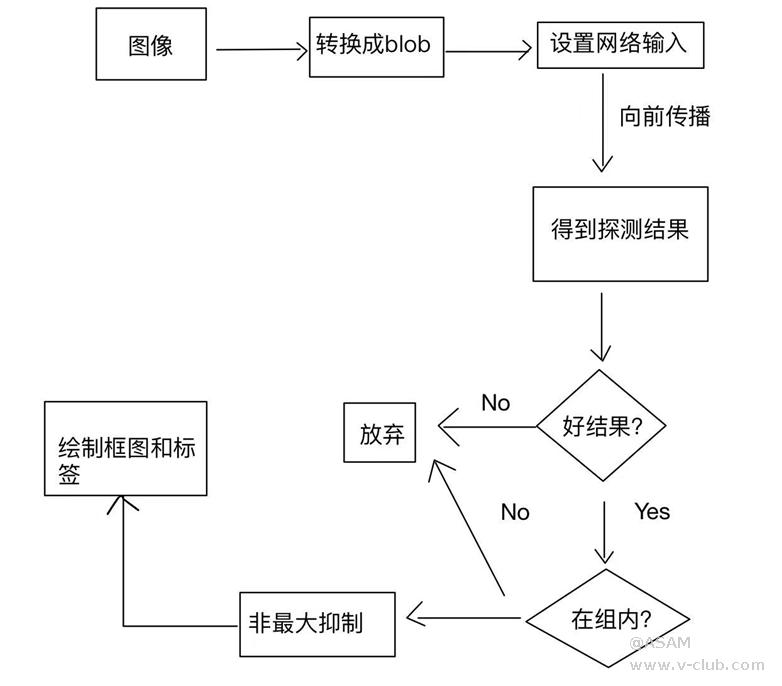## 3.6运行结果

OpenCV版本：       OpenCV3.43

Visual Studio版本：    VS2022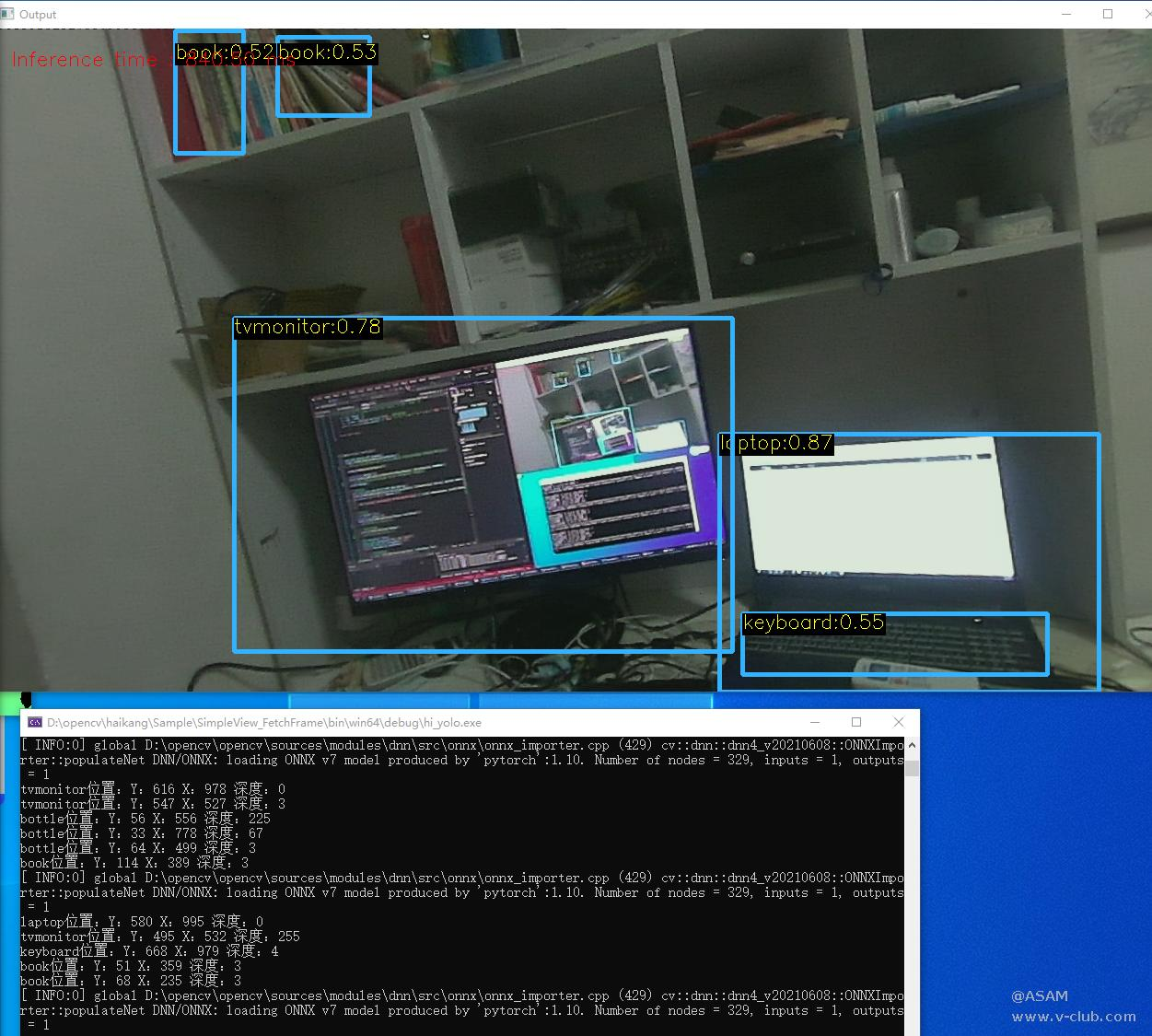•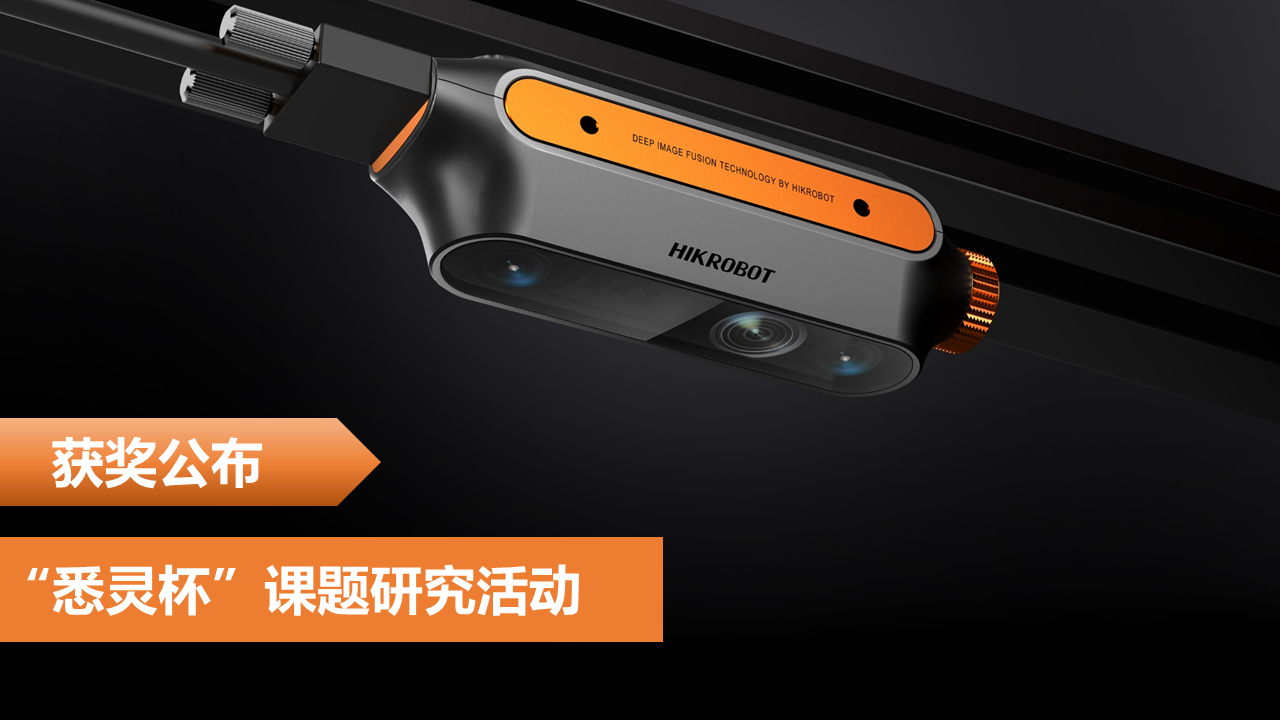海康机器人“悉灵杯”课题研究活动-获奖公布
2022-09-05
•汽车行业-汽车焊点检测案例
2022-09-01
•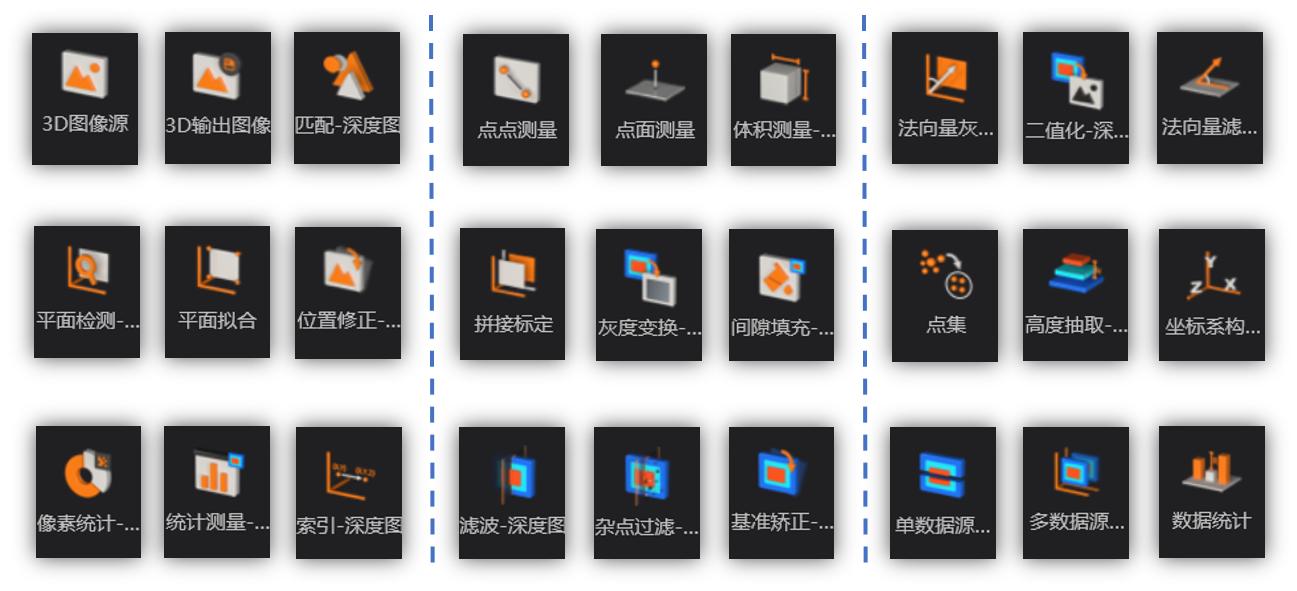VM3D模块学习经验分享
2022-09-22
•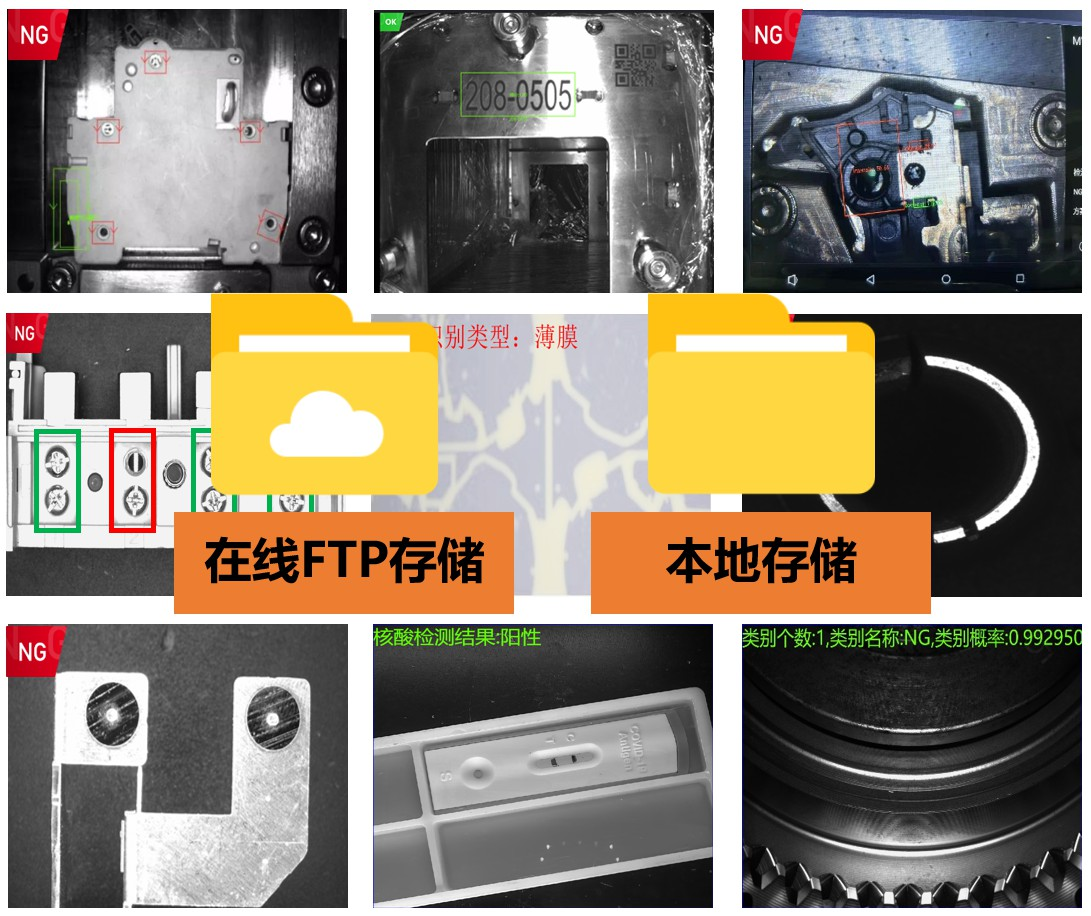SC系列智能相机图像存储的几种方式
2022-09-08
•有关条码二维码相关知识
2022-08-30